CHAPTER 1. Mathematics Solving Proportions Normally when solving a proportion, three quantities will be known, and the fourth will be unknown. To solve for the unknown, multiply the two numbers along the diagonal and then divide by the third number. Example: Solve for X in the proportion given below.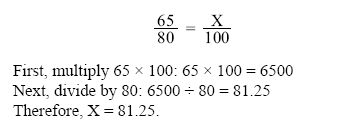Example: An airplane flying a distance of 300 miles used 24 gallons of gasoline. How many gallons will it need to travel 750 miles? The ratio here is: “miles to gallons;" therefore, the proportion is set up as: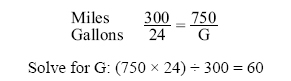Therefore, to fly 750 miles, 60 gallons of gasoline will be required. Percentage Percentage means “parts out of one hundred." The percentage sign is “%". Ninety percent is expressed as 90% (= 90 parts out of 100). The decimal 0.90 equals 90/100, or 90 out of 100, or 90%. Expressing a Decimal Number as a Percentage To express a decimal number in percent, move the decimal point two places to the right (adding zeros if necessary) and then affix the percent symbol. Example: Express the following decimal numbers as a percent: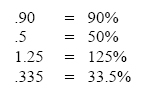Expressing a Percentage as a Decimal Number Sometimes it may be necessary to express a percentage as a decimal number. To express a percentage as a decimal number, move the decimal point two places to the left and drop the % symbol. For example: Express the following percentages as decimal numbers: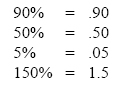Expressing a Fraction as a Percentage To express a fraction as a percentage, first change the fraction to a decimal number (by dividing the numerator by the denominator), and then convert the decimal number to a percentage as shown earlier. Example: Express the fraction 5/8 as a percentage.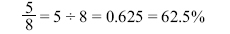Finding a Percentage of a Given Number This is the most common type of percentage calculation. Here are two methods to solve percentage problems: using algebra or using proportions. Each method is shown below to find a percent of a given number. Example: In a shipment of 80 wingtip lights, 15% of the lights were defective. How many of the lights were defective? Algebra Method: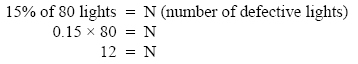Therefore, 12 defective lights were in the shipment. Proportion Method: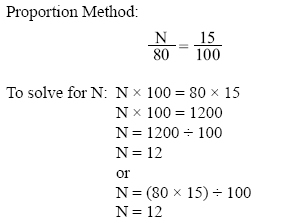Finding What Percentage One Number Is of Another Example: A small engine rated at 12 horsepower is found to be delivering only 10.75 horsepower. What is the motor efficiency expressed as a percent?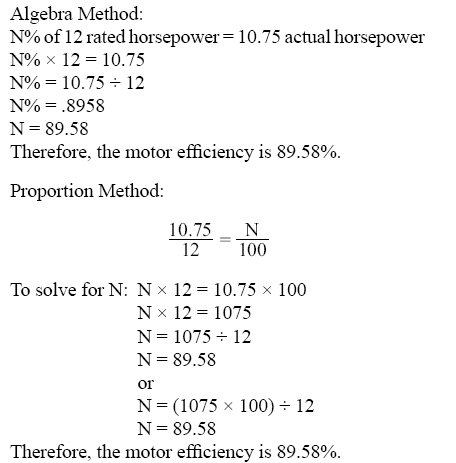©AvStop Online Magazine                                                                                                                                                      Contact Us              Return To Books